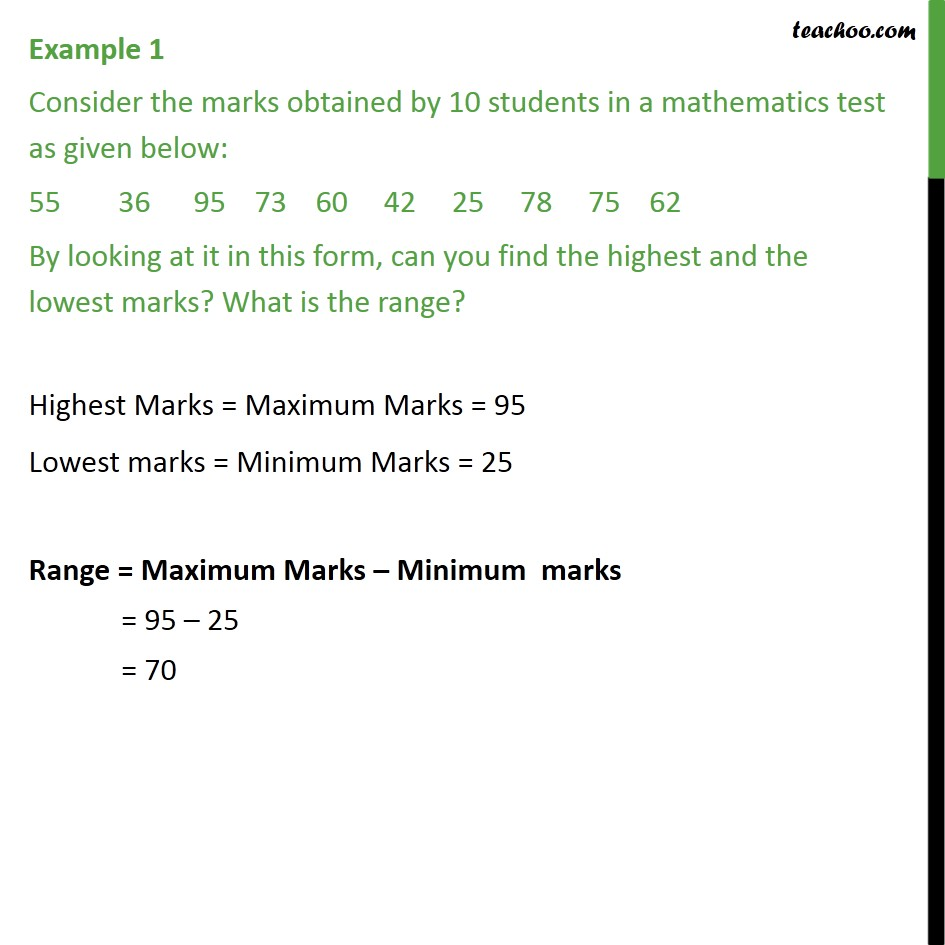1. Chapter 14 Class 9 Statistics (Term 1)
2. Serial order wise
3. Examples

Transcript

Example 1 Consider the marks obtained by 10 students in a mathematics test as given below: 55 36 95 73 60 42 25 78 75 62 By looking at it in this form, can you find the highest and the lowest marks? What is the range? Highest Marks = Maximum Marks = 95 Lowest marks = Minimum Marks = 25 Range = Maximum Marks – Minimum marks = 95 – 25 = 70